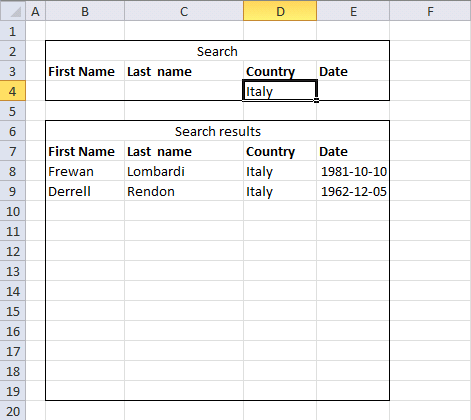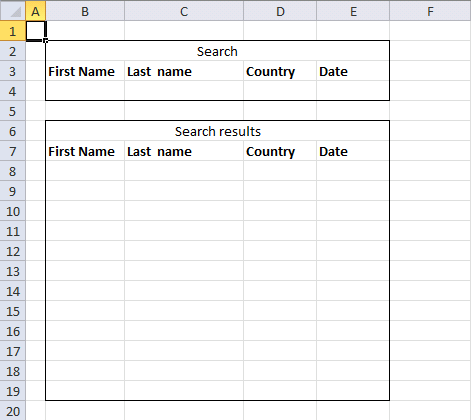Author: Oscar Cronquist Article last updated on December 30, 2018Rashid asks:I used your array formula with great success to find the search results from multiple criteria. However, my problem is modifying your formula. In the above example you have shown us, you have two criteria. And you distinguish the two criteria by using *.

My question is: what would you do if you don't know the predetermined number of criteria. So let's say the person searching only specifies security and not date. Or only date, and not security. Or maybe both. The problem is you don't know from beforehand.
How would you go about solving this problem?

Your help is greatly appreciated, thanks so much!!The data table is on sheet 2.

Array formula in cell B8:

=IFERROR(IF(COUNTA(\$B\$4:\$E\$4)<>0, INDEX(Sheet2!\$A\$2:\$D\$21, SMALL(IF(COUNTA(\$B\$4:\$E\$4)=(COUNTIF(\$B\$4, Sheet2!\$A\$2:\$A\$21)+COUNTIF(Sheet1!\$C\$4, Sheet2!\$B\$2:\$B\$21)+COUNTIF(Sheet1!\$D\$4, Sheet2!\$C\$2:\$C\$21)+COUNTIF(Sheet1!\$E\$4, Sheet2!\$D\$2:\$D\$21)), MATCH(ROW(Sheet2!\$D\$2:\$D\$21), ROW(Sheet2!\$D\$2:\$D\$21)), ""), ROW(A1)), COLUMN(A1)), ""), "")

How to enter an array formula

1. Select cell B8
2. Type the above array formula
3. Press and hold CTRL + SHIFT
4. Press Enter
5. Release all keys

If you got it right, there is now a { before the array formula and a } after the array formula.

### How to copy array formula

1. Copy cell B8
2. Paste to C8:E8
3. Copy cell range B8:E8
4. Paste to cell range B9:E19

### Explaining the array formula in cell B8

Step 1 - Count the number of cells in each table column that meet the criteria and add the arrays

The COUNTIF function counts values based on a condition or criteria.

COUNTIF(\$B\$4, Sheet2!\$A\$2:\$A\$21)+COUNTIF(Sheet1!\$C\$4, Sheet2!\$B\$2:\$B\$21)+COUNTIF(Sheet1!\$D\$4, Sheet2!\$C\$2:\$C\$21)+COUNTIF(Sheet1!\$E\$4, Sheet2!\$D\$2:\$D\$21)

becomes

{0;0;1;0;0;0;0;0;0;0;0;0;0;0;0;0;0;0;0;0})+{0;0;0;0;0;0;0;0;0;0;0;0;0;0;0;0;0;0;0;0}+{0;0;0;0;0;0;0;0;0;0;0;0;0;0;0;0;0;0;0;0}+{0;0;1;0;0;0;0;0;0;0;0;0;0;0;1;0;0;0;0;0}

and returns

{0;0;2;0;0;0;0;0;0;0;0;0;0;0;1;0;0;0;0;0}

This means that there are two criteria matching on row 3 in the table

Step 2 - Count the number of criteria matching and compare with each value in the array above

The IF function has three arguments, the first one must be a logical expression. If the expression evaluates to TRUE then one thing happens (argument 2) and if FALSE another thing happens (argument 3).

IF(COUNTA(\$B\$4:\$E\$4)=(COUNTIF(\$B\$4, Sheet2!\$A\$2:\$A\$21)+COUNTIF(Sheet1!\$C\$4, Sheet2!\$B\$2:\$B\$21)+COUNTIF(Sheet1!\$D\$4, Sheet2!\$C\$2:\$C\$21)+COUNTIF(Sheet1!\$E\$4, Sheet2!\$D\$2:\$D\$21)), MATCH(ROW(Sheet2!\$D\$2:\$D\$21), ROW(Sheet2!\$D\$2:\$D\$21)), "")

becomes

IF(2={0;0;2;0;0;0;0;0;0;0;0;0;0;0;1;0;0;0;0;0}, MATCH(ROW(Sheet2!\$D\$2:\$D\$21), ROW(Sheet2!\$D\$2:\$D\$21)), "")

becomes

IF(2={0;0;2;0;0;0;0;0;0;0;0;0;0;0;1;0;0;0;0;0}, {1;2;3;4;5;6;7;8;9;10;11;12;13;14;15;16;17;18;19;20}, "")

becomes

IF({FALSE; FALSE; TRUE; FALSE; FALSE; FALSE; FALSE; FALSE; FALSE; FALSE; FALSE; FALSE; FALSE; FALSE; FALSE; FALSE; FALSE; FALSE; FALSE; FALSE}, {1;2;3;4;5; 6;7;8;9;10; 11;12;13;14; 15;16;17;18;19;20}, "")

and returns

{"";"";3;""; "";"";"";"";""; "";"";"";"";"";""; "";"";"";"";""}

Step 3 - Find the k-th smallest row number

To be able to return a new value in a cell each I use the SMALL function to filter column numbers from smallest to largest.

SMALL(IF(COUNTA(\$B\$4:\$E\$4)=(COUNTIF(\$B\$4, Sheet2!\$A\$2:\$A\$21)+COUNTIF(Sheet1!\$C\$4, Sheet2!\$B\$2:\$B\$21)+COUNTIF(Sheet1!\$D\$4, Sheet2!\$C\$2:\$C\$21)+COUNTIF(Sheet1!\$E\$4, Sheet2!\$D\$2:\$D\$21)), MATCH(ROW(Sheet2!\$D\$2:\$D\$21), ROW(Sheet2!\$D\$2:\$D\$21)), ""), ROW(A1))

becomes

SMALL({"";"";3;""; "";"";"";"";""; "";"";"";"";"";""; "";"";"";"";""}, ROW(A1))

and returns 3.

Step 4 - Return corresponding value from data table on sheet 2

The INDEX function returns a value based on a cell reference and column/row numbers.

INDEX(Sheet2!\$A\$2:\$D\$21, SMALL(IF(COUNTA(\$B\$4:\$E\$4)=(COUNTIF(\$B\$4, Sheet2!\$A\$2:\$A\$21)+COUNTIF(Sheet1!\$C\$4, Sheet2!\$B\$2:\$B\$21)+COUNTIF(Sheet1!\$D\$4, Sheet2!\$C\$2:\$C\$21)+COUNTIF(Sheet1!\$E\$4, Sheet2!\$D\$2:\$D\$21)), MATCH(ROW(Sheet2!\$D\$2:\$D\$21), ROW(Sheet2!\$D\$2:\$D\$21)), ""), ROW(A1)), COLUMN(A1))

becomes

INDEX(Sheet2!\$A\$2:\$D\$21, 3, COLUMN(A1))

becomes

INDEX(Sheet2!\$A\$2:\$D\$21, 3, 1)

and returns Keisha.

Step 5 - Check if there are more than 0 (zero) citeria

The COUNTA function counts the non-empty or blank cells in a cell range.

COUNTA(\$B\$4:\$E\$4)<>0

becomes

2<>0

and returns TRUE

Step 6 - Return value if there are more than zero criteria

IF(COUNTA(\$B\$4:\$E\$4)<>0, INDEX(Sheet2!\$A\$2:\$D\$21, SMALL(IF(COUNTA(\$B\$4:\$E\$4)=(COUNTIF(\$B\$4, Sheet2!\$A\$2:\$A\$21)+COUNTIF(Sheet1!\$C\$4, Sheet2!\$B\$2:\$B\$21)+COUNTIF(Sheet1!\$D\$4, Sheet2!\$C\$2:\$C\$21)+COUNTIF(Sheet1!\$E\$4, Sheet2!\$D\$2:\$D\$21)), MATCH(ROW(Sheet2!\$D\$2:\$D\$21), ROW(Sheet2!\$D\$2:\$D\$21)), ""), ROW(A1)), COLUMN(A1)), "")

becomes

IF(TRUE, "Keisha", "")

and returns "Keisha" in cell B8.

### Download Excel file

* You will also get a weekly newsletter, unsubscribe anytime!